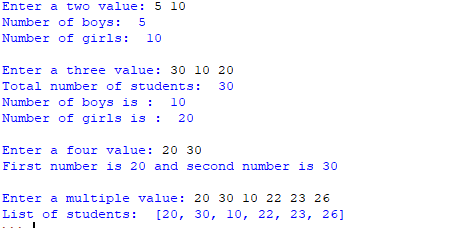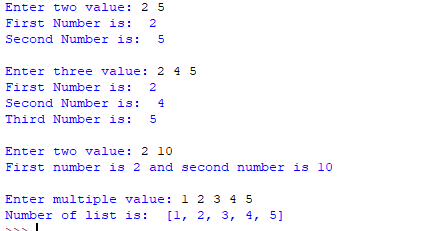# Taking multiple inputs from user in Python

Developer often wants a user to enter multiple values or inputs in one line. In C++/C user can take multiple inputs in one line using scanf but in Python user can take multiple values or inputs in one line by two methods.

• Using split() method
• Using List comprehension

Using split() method :
This function helps in getting a multiple inputs from user. It breaks the given input by the specified separator. If a separator is not provided then any white space is a separator. Generally, user use a split() method to split a Python string but one can use it in taking multiple input.

Syntax :

```input().split(separator, maxsplit)
```

Example :

## Python3

 `# Python program showing how to` `# multiple input using split`   `# taking two inputs at a time` `x, y ``=` `input``(``"Enter a two value: "``).split()` `print``(``"Number of boys: "``, x)` `print``(``"Number of girls: "``, y)` `print``()`   `# taking three inputs at a time` `x, y, z ``=` `input``(``"Enter a three value: "``).split()` `print``(``"Total number of students: "``, x)` `print``(``"Number of boys is : "``, y)` `print``(``"Number of girls is : "``, z)` `print``()`   `# taking two inputs at a time` `a, b ``=` `input``(``"Enter a two value: "``).split()` `print``(``"First number is {} and second number is {}"``.``format``(a, b))` `print``()`   `# taking multiple inputs at a time ` `# and type casting using list() function` `x ``=` `list``(``map``(``int``, ``input``(``"Enter a multiple value: "``).split()))` `print``(``"List of students: "``, x)`

Output:Using List comprehension :
List comprehension is an elegant way to define and create list in Python. We can create lists just like mathematical statements in one line only. It is also used in getting multiple inputs from a user.

Example:

## Python3

 `# Python program showing` `# how to take multiple input` `# using List comprehension`   `# taking two input at a time` `x, y ``=` `[``int``(x) ``for` `x ``in` `input``(``"Enter two value: "``).split()]` `print``(``"First Number is: "``, x)` `print``(``"Second Number is: "``, y)` `print``()`   `# taking three input at a time` `x, y, z ``=` `[``int``(x) ``for` `x ``in` `input``(``"Enter three value: "``).split()]` `print``(``"First Number is: "``, x)` `print``(``"Second Number is: "``, y)` `print``(``"Third Number is: "``, z)` `print``()`   `# taking two inputs at a time` `x, y ``=` `[``int``(x) ``for` `x ``in` `input``(``"Enter two value: "``).split()]` `print``(``"First number is {} and second number is {}"``.``format``(x, y))` `print``()`   `# taking multiple inputs at a time ` `x ``=` `[``int``(x) ``for` `x ``in` `input``(``"Enter multiple value: "``).split()]` `print``(``"Number of list is: "``, x) `

Output :Note : The above examples take input separated by spaces. In case we wish to take input separated by comma (, ), we can use following:

## Python3

 `# taking multiple inputs at a time separated by comma` `x ``=` `[``int``(x) ``for` `x ``in` `input``(``"Enter multiple value: "``).split(``","``)]` `print``(``"Number of list is: "``, x) `

Please see https://ide.geeksforgeeks.org/BHf0Cxr4mx for a sample run.

My Personal Notes arrow_drop_upIf you like GeeksforGeeks and would like to contribute, you can also write an article using contribute.geeksforgeeks.org or mail your article to contribute@geeksforgeeks.org. See your article appearing on the GeeksforGeeks main page and help other Geeks.

Please Improve this article if you find anything incorrect by clicking on the "Improve Article" button below.

Article Tags :

61

Please write to us at contribute@geeksforgeeks.org to report any issue with the above content.